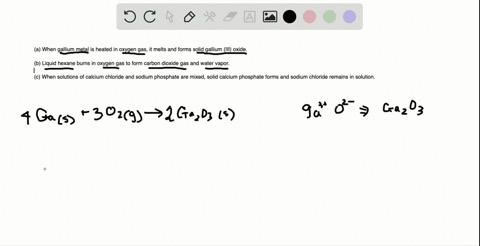Black Friday is Here! Start Your Numerade Subscription for 50% Off!Join Today### Convert the following into balanced equations: (…

07:28University of Toronto
Problem 59

# Write balanced equations for each of the following by inserting the correct coefficients in the blanks:(a) $_{-}\mathrm{As}_{4} \mathrm{S}_{6}(s)+{_{-}\mathrm{O}_{2}(g)} \longrightarrow_{-}\mathrm{As}_{4} \mathrm{O}_{6}(s)+_{-}\mathrm{SO}_{2}(g)$(b) $_{-}\mathrm{Ca}_{3}\left(\mathrm{PO}_{4}\right)_{2}(s)+_{-}\mathrm{SiO}_{2}(s)+_{-}\mathrm{C}(s) \longrightarrow _{-}\mathrm{P}_{4}(g)+_{-}\mathrm{CaSiO}_{3}(l)+_{-}\mathrm{CO}(g)$(c) $_{-}\mathrm{Fe}(s)+_{-}\mathrm{H}_{2} \mathrm{O}(g) \longrightarrow-_{-}\mathrm{Fe}_{3} \mathrm{O}_{4}(s)+_{-}\mathrm{H}_{2}(g)$(d) $_{-}\mathrm S_{2} \mathrm{Cl}_{2}(l)+_{-}\mathrm{NH}_{3}(g) \longrightarrow_{-} \mathrm{S}_{4} \mathrm{N}_{4}(s)+_{-}\mathrm{S}_{8}(s)+_{-}\mathrm{NH}_{4} \mathrm{Cl}(s)$

## Discussion

You must be signed in to discuss.